## A ball is thrown upward with a speed of 40 m/s. Approximately how much time goes it take the ball to travel from the release location

Question

A ball is thrown upward with a speed of 40 m/s. Approximately how much time goes it take the ball to travel from the release location (A) to its highest point(B)? Approximately how much total time is the ball in the air before it returns to back to its original height (C)?

in progress 0
6 months 2021-07-17T12:23:15+00:00 1 Answers 13 views 0

(I). The time at highest point 4.0 sec.

(II).  It returns to back to its original height in 8.1 sec

Explanation:

Given that,

Velocity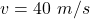(I). We need to calculate the time at highest point

Using equation of motion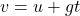Where, v = final velocity

u = initial velocity

g = acceleration due to gravity

t = time

Put the value into the formula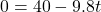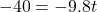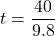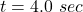(II). We know that, when the ball to travel from the initial point and reached at initial point then the displacement is zero.

We need to calculate the total time when it returns to back to its original height

Using equation of motion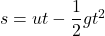Where, s = displacement

g = acceleration due to gravity

t = time

u = velocity

Put the value in the equation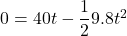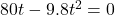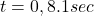Hence. (I). The time at highest point 4.0 sec.

(II). It returns to back to its original height in 8.1 sec